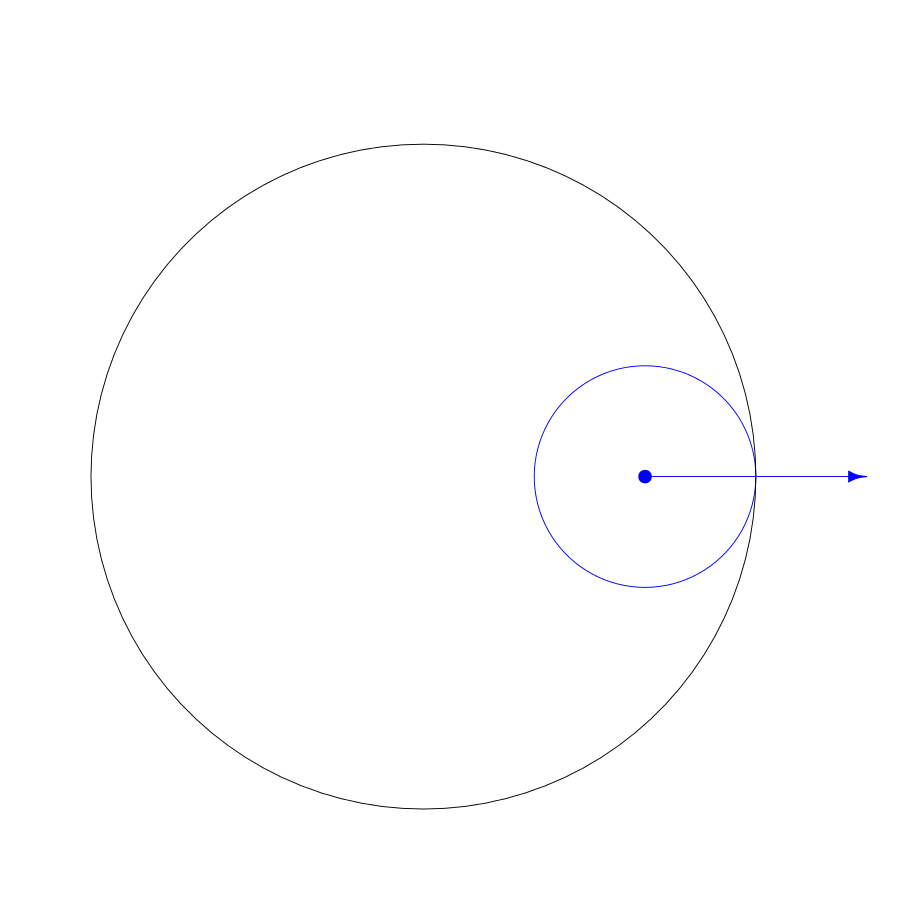As a kid, I loved making spirographs. I still do. Making them feels more like discovery than creativity, like finding hidden wings in the Mathematical Museum of Art. I have not yet found the point where spirographs no longer surprise me.

The surprising variety of forms generated by spirographs are manifestations of just one equation, the circular path troichoid. The shape of the spirograph depends on the radius of a fixed circle, radius of a cycling circle, and the distance of the pen from the center of the the cycling circle.

\begin{align} x (\theta) &= (R - r)\cos\theta + d\cos\left({R - r \over r}\theta\right)\\ y (\theta) &= (R - r)\sin\theta - d\sin\left({R - r \over r}\theta\right) \end{align}

Where
R is the radius of the fixed circle
r is the radius of the cycling circle
d is the distance of the pen from the center of the cycling circle
θ is the number of radians the cycling circle travels around the fixed circle
x(θ) is the position of x after the cycling circle travels θ radians
y(θ) is the position of y after the cycling circle travels θ radians

cycling_radius <- 1

d_circle <- tibble(
y0 = c(0, 0),
r_y = c(0, 0),
color = c("black", "royalblue")
)

d_segment <- tibble(
y = c(0, 0, 0),
yend = c(0, 0, 0),
color = c("black", "royalblue", "firebrick")
)

ggplot(data = d_circle) +
theme_void() +
ggforce::geom_circle(
aes(
x0 = x0,
y0 = y0,
color = color),
n = 1000) +
coord_equal() +
geom_text(
aes(
x = r_x,
y = r_y,
label = r_lab,
color = color),
vjust = 0.5,
nudge_y = 0.015,
angle = 0) +
annotate(
y = 0.015,
geom = "label",
color = "firebrick",
label = "Pen\nDistance",
label.size = 0,
geom_segment(
data = d_segment,
aes(x = x, y = y, xend = xend, yend = yend, color = color),
geom = "segment",
linejoin = "mitre",
arrow = arrow(
length = unit(0.025, "npc"),
type = "closed",
angle = 15)) +
annotate(
y = 0,
geom = "point",
color = "royalblue") +
scale_color_identity() +
theme(legend.position = "none")Three parameters of spirograph shapesIn this spirograph,
the fixed radius R is 3,
the cycling radius r is 1,
and the pen radius d is 2.

Although I still like making spirographs by hand, I wanted to extend what could be done with the traditional spirograph. I wrote the spiro package in R to make images that would be impossible to create on paper.

I cannot usually predict what will happen when I play with the three primary numbers of the equation. However, once a certain combination strikes me as interesting, I play with cutting it into different color segments to see if something of further interest happens. Sometimes I merge many spirographs and spin them to see if the emerging patterns are pleasing.

Here I demonstrate what can be done with spiro package. I would love to see what you can do with it.

# Welcome Everyone

spiro(
colors = viridis::viridis(67),
color_groups = 67,
color_cycles = 59,
windings = 96,
points_per_polygon = 50,
file = "viridis_weave.svg"
) %>%
add_background(color = "gray8")# My Non-Canonical Backstory

k <- 8
rowid_to_column("id") %>%
mutate(
colors = lacroix_palette("Coconut", n = k , "continuous"),
file = paste0("sdfds.", id, ".svg")
) %>%
select(-id) %>%
pmap(
spiro,
points_per_polygon = 2000,
draw_fills = F,
transparency = 0.9) %>%
image_merge(
output = "my_non_canonical_backstory.svg") %>%
add_background()# Licorice Donut Vivisection

rainbow_colors <- hsv(
h = seq(1 / 16, 1, length.out = 16),
s = 0.7,
v = 0.7)

spiro(
file = "licorice_donut_vivisection.svg",
color_groups = 16,
color_cycles = 2,
points_per_polygon = 50,
colors = rainbow_colors,
transparency = 0.7) %>%
colors = c("white", "black", "black", "white"),
stops = c(.27, .34, .93, 1),
rounding = 1,
radius = 1)# When We Meet Again,Will We Be As We Were?

n <- 10
oslo_colors <- scico(
n = n,
palette = "oslo",
alpha = 0.9) %>%
rev()

spiro(
file = "oslo_aster.svg",
rotation = pi / 6,
points_per_polygon = 100) %>%
image_merge(
output = "oslo_aster.svg",
copies = n) %>%
colors = oslo_colors) %>%
image_scale(
scale = sqrt(0.75 ^ (seq(0, n - 1)))) %>%
image_spin(
rpm = 1:n + 1) %>%
color = "black",
rounding = 1) %>%
add_restart()

# But for the DarknessNothing Shimmers

set.seed(105)
k <- 15
bg_colors <- paste0("gray", sample(1:k, k))

bg_stops <- sort(runif(k))

spiro(
fixed_radius = 2 * 13 * 17,
cycling_radius = 3 * 11 * 19,
file = "but_for_the_darkness_nothing_shimmers.svg",
draw_fills = F,
line_width = 3,
color_groups = 380,
color_cycles = 31,
points_per_polygon = 100,
colors = c(
scico(60 * 2, palette = "lisbon", 0.8),
scico(40 * 2, palette = "cork", 0.25),
scico(20 * 2, palette = "lisbon", 1))) %>%
add_background_gradient(rounding = 0, colors = bg_colors)# You’re My Favorite

k <- 36
files <- paste0("s", 1:k, ".svg")
pen_radii <- seq(3.8, 1.5, length.out = k)
alphas <- rep_len(c(0.85, rep(0.2, 4)), k)
colors <- rep_len(scico(6, palette = "devon"), k) %>%
alpha(., alpha = alphas)

tibble::tibble(
file = files,
colors = colors) %>%
purrr::pmap_chr(
spiro,
rotation = -pi / 10,
points_per_polygon = 500,
draw_fills = T,
xlim = c(-7, 7),
ylim = c(-7, 7)) %>%
image_merge(
output = "youre_my_favorite.svg") %>%
add_lines(colors = c(rep(NA,k - 1), "gray")) %>%
image_rotate(degrees = (1:k / 2.5)) %>%
colors = c(
"#FFFFFF",
"#26588E",
"#E5E3F9",
"#283568",
"#C8C3F3"),
rounding = 1,
stops = c(0.42,0.93,0.96,0.97,1))# Suspension of Disbelief

n <- 20
spiro(
add_restart()